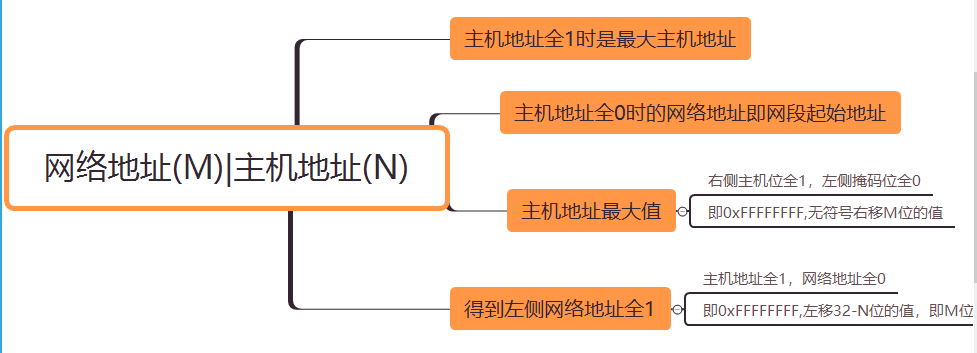• IP/掩码位来表示一段IP范围，那么怎么通过掩码位计算出对应的子网掩码呢？基本概念：掩码位即网络地址的位数。例如192.168.11.130/31表示网络地址有31位，主机地址有1位，那么31对应的子网掩码就是255.255.255.254...
IP/掩码位来表示一段IP范围，那么怎么通过掩码位计算出对应的子网掩码呢？基本概念：掩码位即网络地址的位数。例如192.168.11.130/31表示网络地址有31位，主机地址有1位，那么31对应的子网掩码就是255.255.255.254(31个1+0)计算掩码位，就是得到掩码位的二进制序列，然后每隔8位转换为十进制。Java的Integer.parseInt可以将指定进制的字符串序列转换为int类型。输入一个掩码位，得到对应的子网掩码的点分十进制表示的代码如下：for(int i=1;i<32;i++) {int ip = 0xFFFFFFFF << (32 - i);String binaryStr = Integer.toBinaryString(ip);StringBuffer buffer = new StringBuffer();for(int j=0;j<4;j++) {int beginIndex = j*8;buffer.append(Integer.parseInt(binaryStr.substring(beginIndex, beginIndex+8), 2)).append(".");}System.out.println("net mask "+i+" ,submask:"+buffer.substring(0,buffer.length()-1));}上述代码即可输出1-31掩码位对应的子网掩码列表。其中0xFFFFFFFF << (32 - i)就是得到对应的二进制序列。
展开全文• IP/掩码位来表示一段IP范围，那么怎么通过掩码位计算出对应的子网掩码呢？基本概念：掩码位即网络地址的位数。例如192.168.11.130/31表示网络地址有31位，主机地址有1位，那么31对应的子网掩码就是255.255.255.254...
IP/掩码位来表示一段IP范围，那么怎么通过掩码位计算出对应的子网掩码呢？基本概念：掩码位即网络地址的位数。例如192.168.11.130/31表示网络地址有31位，主机地址有1位，那么31对应的子网掩码就是255.255.255.254（31个1+0）通过移位计算，得到主机最大值，和网络地址最大值，图解原理如下：计算掩码位，就是得到掩码位的二进制序列，然后每隔8位转换为十进制。Java的Integer.parseInt可以将指定进制的字符串序列转换为int类型。输入一个掩码位，得到对应的子网掩码的点分十进制表示的代码如下：for(int i=1;i<32;i++) {
int ip = 0xFFFFFFFF << (32 - i);
String binaryStr = Integer.toBinaryString(ip);
StringBuffer buffer = new StringBuffer();
for(int j=0;j<4;j++) {
int beginIndex = j*8;
buffer.append(Integer.parseInt(binaryStr.substring(beginIndex, beginIndex+8), 2)).append(".");
}
System.out.println("net mask "+i+" ,submask:"+buffer.substring(0,buffer.length()-1));

}上述代码即可输出1-31掩码位对应的子网掩码列表。其中0xFFFFFFFF << (32 - i)就是得到对应的二进制序列。
展开全文• ip2long IP地址转换成整型。long2ip 整型数据转换成IP。子网掩码转换成掩码长度方式：$slash_notation = strlen(preg_replace("/0/", "", decbin(ip2long($subnet_mask))));$bits=strpos(decbin(ip2long($mask)),"0...
ip2long IP地址转换成整型。long2ip 整型数据转换成IP。子网掩码转换成掩码长度方式：$slash_notation = strlen(preg_replace("/0/", "", decbin(ip2long($subnet_mask))));$bits=strpos(decbin(ip2long($mask)),"0");子网掩码位长转换成子网掩码形式：$mask = 0xffffffff << (32 -$mask);$mask = pow(2,32)-pow(2,(32-$mask));判断两个地址是否在一个子网内：function matchCIDR($addr,$cidr) {list($ip,$mask) = explode('/', $cidr);return (ip2long($addr) >> (32 - $mask) == ip2long($ip) >> (32 - mask));}?>一个IPv4的类：//--------------// IPv4 classclass ipv4{var $address;var$netbits;//--------------// Create new classfunction ipv4($address,$netbits){$this->address =$address;$this->netbits =$netbits;}//--------------// Return the IP addressfunction address() { return ($this->address); }//--------------// Return the netbitsfunction netbits() { return ($this->netbits); }//--------------// Return the netmaskfunction netmask(){return (long2ip(ip2long("255.255.255.255")<< (32-$this->netbits)));}//--------------// Return the network that the address sits infunction network(){return (long2ip((ip2long($this->address))& (ip2long($this->netmask()))));}//--------------// Return the broadcast that the address sits infunction broadcast(){return (long2ip(ip2long($this->network())| (~(ip2long($this->netmask())))));}//--------------// Return the inverse mask of the netmaskfunction inverse(){return (long2ip(~(ip2long("255.255.255.255")<< (32-$this->netbits))));}}$ip = new ipv4("192.168.2.1",24);print "Address:$ip->address()\n";print "Netbits: $ip->netbits()\n";print "Netmask:$ip->netmask()\n";print "Inverse: $ip->inverse()\n";print "Network:$ip->network()\n";print "Broadcast: $ip->broadcast()\n";?>这个做法比较有创意：For those poor little people using PHP 3, here's an ip2long:if (!function_exists("ip2long")) {function ip2long($ip) {$ex = explode(".",$ip);if (count($ex)!=4) return -1;list($a, $b,$c, $d) =$ex;$a =$a*16777216;$b =$b*65536;$c =$c*256;return $a+$b+$c+$d;}}?>#!/usr/local/bin/php$ip_addr = "172.14.1.57";$subnet_mask = "255.255.255.0";$ip = ip2long($ip_addr);$nm = ip2long($subnet_mask);$nw = ($ip & $nm);$bc = $nw | (~$nm);echo "IP Address:         " . long2ip($ip) . "\n";echo "Subnet Mask: " . long2ip($nm) . "\n";echo "Network Address:    " . long2ip($nw) . "\n";echo "Broadcast Address: " . long2ip($bc) . "\n";echo "Number of Hosts:    " . ($bc -$nw - 1) . "\n";echo "Host Range:         " . long2ip($nw + 1) . " -> " . long2ip($bc - 1)  . "\n";?>Produces the output:IP Address:         172.14.1.57Subnet Mask:        255.255.255.0Network Address:    172.14.1.0Broadcast Address:  172.14.1.255Number of Hosts:    254Host Range:         172.14.1.1 -> 172.14.1.254
展开全文• PHP对IP地址和子网掩码的处理方法(转)(2011-07-15 11:33:36)标签：子网掩码ipv4ip地址数据转换处理方法it分类：phpip2long IP地址转换成整型。long2ip 整型数据转换成IP。子网掩码转换成掩码长度方式：$slash_... PHP对IP地址和子网掩码的处理方法(转)(2011-07-15 11:33:36)标签：子网掩码ipv4ip地址数据转换处理方法it分类：phpip2long IP地址转换成整型。long2ip 整型数据转换成IP。子网掩码转换成掩码长度方式：$slash_notation =strlen(preg_replace("/0/", "",decbin(ip2long($subnet_mask))));$bits=strpos(decbin(ip2long(...ip2long IP地址转换成整型。long2ip 整型数据转换成IP。子网掩码转换成掩码长度方式：$slash_notation = strlen(preg_replace("/0/", "",decbin(ip2long($subnet_mask))));$bits=strpos(decbin(ip2long($mask)),"0");子网掩码位长转换成子网掩码形式：$mask = 0xffffffff << (32 -$mask);$mask = pow(2,32)-pow(2,(32-$mask));判断两个地址是否在一个子网内：function matchCIDR($addr,$cidr) {list($ip,$mask) = explode('/', $cidr);return (ip2long($addr) >> (32 -$mask) == ip2long($ip) >> (32 -mask));}?>一个IPv4的类：//--------------// IPv4 classclass ipv4{var $address;var$netbits;//--------------// Create new classfunction ipv4($address,$netbits){$this->address =$address;$this->netbits =$netbits;}//--------------// Return the IP addressfunction address() { return ($this->address);}//--------------// Return the netbitsfunction netbits() { return ($this->netbits);}//--------------// Return the netmaskfunction netmask(){return (long2ip(ip2long("255.255.255.255")<<(32-$this->netbits)));}//--------------// Return the network that the address sits infunction network(){return (long2ip((ip2long($this->address))&(ip2long($this->netmask()))));}//--------------// Return the broadcast that the address sits infunction broadcast(){return (long2ip(ip2long($this->network())| (~(ip2long($this->netmask())))));}//--------------// Return the inverse mask of the netmaskfunction inverse(){return (long2ip(~(ip2long("255.255.255.255")<<(32-$this->netbits))));}}$ip = new ipv4("192.168.2.1",24);print "Address:$ip->address()\n";print "Netbits: $ip->netbits()\n";print "Netmask:$ip->netmask()\n";print "Inverse: $ip->inverse()\n";print "Network:$ip->network()\n";print "Broadcast: $ip->broadcast()\n";?>这个做法比较有创意：For those poor little people using PHP 3, here's an ip2long:if (!function_exists("ip2long")) {function ip2long($ip) {$ex = explode(".",$ip);if (count($ex)!=4) return -1;list($a, $b,$c, $d) =$ex;$a =$a*16777216;$b =$b*65536;$c =$c*256;return $a+$b+$c+$d;}}?>#!/usr/local/bin/php$ip_addr = "172.14.1.57";$subnet_mask = "255.255.255.0";$ip = ip2long($ip_addr);$nm = ip2long($subnet_mask);$nw = ($ip & $nm);$bc = $nw | (~$nm);echo "IP Address: " . long2ip($ip) . "\n";echo "Subnet Mask: " . long2ip($nm) . "\n";echo "Network Address: " . long2ip($nw) . "\n";echo "Broadcast Address: " . long2ip($bc) . "\n";echo "Number of Hosts: " . ($bc -$nw - 1) . "\n";echo "Host Range: " . long2ip($nw + 1) . " -> " .long2ip($bc - 1) . "\n";?>Produces the output:IP Address: 172.14.1.57Subnet Mask: 255.255.255.0Network Address: 172.14.1.0Broadcast Address: 172.14.1.255Number of Hosts: 254Host Range: 172.14.1.1 ->172.14.1.254分享：喜欢0赠金笔加载中，请稍候......评论加载中，请稍候...发评论登录名：   密码：   找回密码   注册记住登录状态昵   称：评论并转载此博文发评论以上网友发言只代表其个人观点，不代表新浪网的观点或立场。
展开全文• IP 地址IP 32位，分为网络号和...如何根据IP地址子网掩码，计算网络地址：①、将IP地址子网掩码转换成二进制数。②、将二进制形式的 IP 地址与子网掩码做“与”运算。③、将得出的结果转化为十进制，便得到网...
• IP地址子网掩码换算 已知ip地址和子网位数，例如：C网192.168.1.53/27，求（1）具体的子网掩码（2）子网数（3）最大可容纳主机数（4）可用的主机数 （5）网络地址（6）广播地址（7）地址范围 （8）主机号 一...
• IP地址子网掩码 1、IP地址 1）IP地址形式 四个十进制数用 . 分隔，计算机使用时会将每个十进制数转换成8bit的二进制数，所以IP地址使用二进制表示为32bit。 2）IP地址的组成 网络地址（网络位）：子网的地址编号...运维
• ip2long IP地址转换成整型。long2ip 整型数据转换成IP。子网掩码转换成掩码长度方式：$slash_notation = strlen(preg_replace("/0/", "", decbin(ip2long($subnet_mask))));$bits=strpos(decbin(ip2long($mask)),"0...
• 一直以来对于IP地址的分类和子网掩码的作用比较模糊，总是感觉似懂非懂，现在主要将其进行整理，抛开一些很深邃的技术概念，单纯对基本知识进行整理。 一、IP地址的分类：  目前IP地址被分为A、B、C、D、E五种...网络基础知识
• 子网掩码转换为IP范围   在网络应用中,经常需要将子网...一 子网掩码的作用, 就是将某个IP地址划分为'子网编号'和'主机地址'  掩码格式 [子网编号:26bit ]主机地址:6bit  172.16.2.64/26 [10101100 00010000 00网络
• 计算机网络考试中一般会给你一个IP地址，计算其子网掩码 例如牛客网的这道题 做题思路步骤如下： 首先知道网络位号共23位，32-23=9； 所以二进制表示：11111111 11111111 11111110 0000 0000 计算将该二进制...计算机网络
• 初学者往往搞不清网段划分，更不知道该如何配置子网掩码，今天我来给小白们扫扫盲。 太理论的我今天不想讲，只想结合实例，说明一下，网段的划分。 在阐述正题之前，我们先来说一下10进制，2进制，因为这个问题，要...
• 子网掩码只有一个作用，就是将某个IP地址划分网络地址和主机地址两部分。子网掩码的作用通过 IP 地址的二进制与子网掩码的二进制进行与运算，确定某个设备的网络地址和主机号，也就是说通过子网掩...
• 在Internet上域名与IP地址之间是一一对应的，域名虽然便于人们记忆，但机器之间只能互相认识IP地址，它们之间的转换工作称为域名解析，域名解析需要由专门的域名解析服务器来完成，DNS就是进行域名解析的服务器 。...
• 子网掩码：判断2个ip地址是不是在同一个网段，在同一个网段的2个地址，可以直接通信。 默认网关：数据包默认选择的出口，一般是路由器地址 DNS：把网站的域名转换成计算机能看懂的ip地址   ...
• 计算：将IP地址子网掩码转换为二进制的形式，然后进行“与”计算。得到网络号，也就是该子网的首地址。 一直纠结于为什么这么计算，现在整理下对这个计算的理解： 1、  首先是子网掩码可以根据子网中的主机数...网络
• 作用：用来发送接收数据的，将模拟信号转换成数字信号 mac地址：每块网卡都有一个全球唯一的标识ID：这个全球唯一的标识ID号 就是所说的mac地址（物理地址 48b 6字节） ip地址：用来标识主机或网卡的一个虚拟ip ip...
• 子网掩码只有一个作用，就是将某个IP地址划分网络地址和主机地址两部分。子网掩码的作用通过 IP 地址的二进制与子网掩码的二进制进行与运算，确定某个设备的网络地址和主机号，也就是说通过子网掩...
• 1.IP地址 在网络中，所有的设备都会被分配一个地址。这个地址就相当于某条路上的...IP地址用十进制表示，由于计算机只能识别二进制，所以在计算机中会转换成二进制的形式。 如：(十进制IP地址) 14.145.184.184 ...
• IP转换成二进制： 00001010 10010001 10000001 00010010 掩码转换成二进制： 11111111 11111111 11111000 00000000 IP掩码相与得网络地址（全1为1，见0为0）：...网络地址转换成十进制为：10,145,128,0 看你的网络地址 广播地址
• ipv4地址是32位的因为ip地址为xxx.xxx.xxx.xxx的形式，每一组被.分割，xxx为0-255之间，所以我们转换成2进制的话为8位。 8*4=32位ipv4地址xxx.xxx.xxx.xxx的这种形式有几个含义 有子网和主机id组成，子网位数可能有...
• 为便于记忆把二进制转换成十进制（210.73.140.6） IP地址形式（10进制）： x.x.x.x x的范围: 0-255 三.子网掩码 局域网通信规则：在同一个局域网中，所有IP必须在同一个网段才可以互相通信 IP构成：网络位 +主机...信息安全
• 废话不多说了直接上程序 ...unsigned int sysconvert(unsigned int x) //实现将十进制转换成二进制 { int b,k,r,i; k=-1; do { r=x%2; b[++k]=r; x/=2; }while(x>=1); for(i=k;i>=0;i--) printf(c语言 1024程序员节
• 192.168.1.1转换成二进制 11000000 10101000 00000001 00000001 子网掩码可以看出来有多少位是主机号 多少位是网络号 例如： 255.255.255.0 11111111 11111111 11111111 00000000 网络号24位 ，全部是1 。 主机...
• 在Internet上域名与IP地址之间是一一对应的，域名虽然便于人们记忆，但机器之间只能互相认识IP地址，它们之间的转换工作称为域名解析，域名解析需要由专门的域名解析服务器来完成，DNS就是进行域名解析的服务器 。...DNS服务器
• 1）将ip地址子网掩码转换成二进制；  2）将二进制形式的ip地址子网掩码做’与’运算，将答案化为十进制便得到网络地址；  3）将二进制形式的子网掩码取’反’；  4）将取’反’后的子网掩
• 在Internet上域名与IP地址之间是一一对应的，域名虽然便于人们记忆，但机器之间只能互相认识IP地址，它们之间的转换工作称为域名解析，域名解析需要由专门的域名解析服务器来完成，DNS就是进行域名解析的服务器 。......